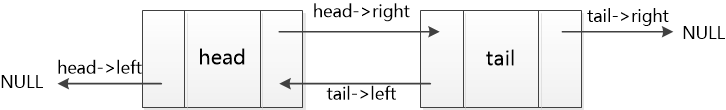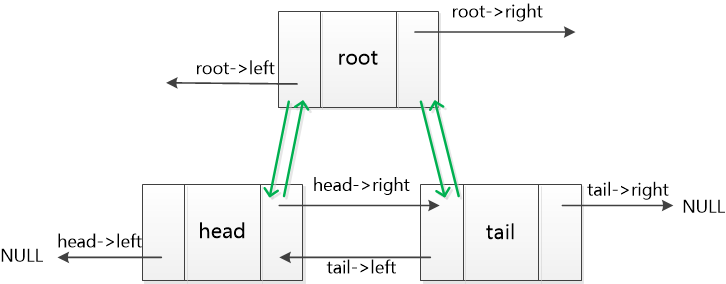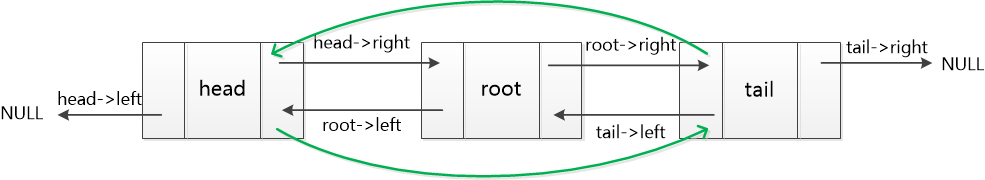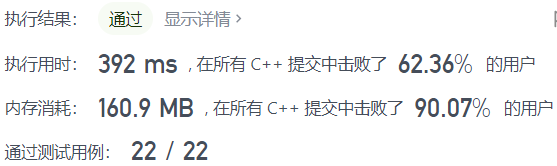# leedcode 146. LRU 缓存机制（哈希+双向链表）

## 题目描述

LRUCache(int capacity) 以正整数作为容量 capacity 初始化 LRU 缓存
int get(int key) 如果关键字 key 存在于缓存中，则返回关键字的值，否则返回 -1 。
void put(int key, int value) 如果关键字已经存在，则变更其数据值；如果关键字不存在，则插入该组「关键字-值」。当缓存容量达到上限时，它应该在写入新数据之前删除最久未使用的数据值，从而为新的数据值留出空间。

## 示例

["LRUCache", "put", "put", "get", "put", "get", "put", "get", "get", "get"]
[, [1, 1], [2, 2], , [3, 3], , [4, 4], , , ]

[null, null, null, 1, null, -1, null, -1, 3, 4]

LRUCache lRUCache = new LRUCache(2);
lRUCache.put(1, 1); // 缓存是 {1=1}
lRUCache.put(2, 2); // 缓存是 {1=1, 2=2}
lRUCache.get(1);    // 返回 1
lRUCache.put(3, 3); // 该操作会使得关键字 2 作废，缓存是 {1=1, 3=3}
lRUCache.get(2);    // 返回 -1 (未找到)
lRUCache.put(4, 4); // 该操作会使得关键字 1 作废，缓存是 {4=4, 3=3}
lRUCache.get(1);    // 返回 -1 (未找到)
lRUCache.get(3);    // 返回 3
lRUCache.get(4);    // 返回 4

1 <= capacity <= 3000
0 <= key <= 10000
0 <= value <= 105

## 题解

• 哈希+双向链表
• 这题是我面试字节跳动实习遇到的题，当时很快想到了哈希，之后在面试官的层层要求 O(1) 时间复杂度进行优化下，想到了队列，也想到了链表，但是也没有完全想明白双向链表。这次，花了一些时间，自己想到了双向链表，哈希方法也从原来两个set和一个map，自己优化到了一个map。
• get o(1) 挺好想的，就一个哈希，就是 put 操作o(1)，就需要链表。然后，考虑到 LRU ，每次 get 过或者 put 的「关键字-值」，就要把它放到链表尾，那么最近最少访问的节点就会自然而然在头节点的位置。缓存满的时候，就要把和头节点连接的那个节点删掉。
• map 映射可以是链表中的一个节点  unordered_map<int,node*>node_mp;//key与一个链表中的节点形成映射
• 注意双向链表的插入、删除，本题为了方便删除第一个节点和插入最后一个节点，我用了头指针和尾指针。一开始初始情况是这样。• 后面如何插入节点的示意图，插入到尾部和删除头部可以自己画画。都是双向链表操作的基础知识。• c++ map删除元素的三种方式

//删除键为bfff指向的元素
cmap.erase("bfff");

//删除迭代器 key所指向的元素
map<string,int>::iterator key = cmap.find("mykey");
if(key!=cmap.end())
{
cmap.erase(key);
}

//删除所有元素
cmap.erase(cmap.begin(),cmap.end())


class LRUCache {
public:
int n;//缓存容量
int count;//缓存中已有多少 「关键字-值」
struct node{
int key;//存储「关键字-值」
int value;
node *left;
node *right;
};
unordered_map<int,node*>node_mp;//key与一个链表中的节点形成映射

node *tail = new node();//全局变量尾节点

LRUCache(int capacity) {
n=capacity;//设置缓存长度赋值全局变量n
count=0;

tail->right=nullptr;
}

int get(int key) {

if(node_mp.count(key)) {//如果存在

node *root=node_mp[key];//将这个节点从原来的地方删除
root->left->right=root->right;
root->right->left=root->left;

root->right=tail;//再把这个节点插到链表尾部
root->left=tail->left;
tail->left->right=root;
tail->left=root;
node_mp[key]=root;//重新与新节点映射
return root->value;//返回value值
}
else return -1;
}

void put(int key, int value) {

if(node_mp.count(key)) {//如果在缓存中

node *root=node_mp[key];//将这个节点从原来的地方删除
root->key=key;//可能需要重新赋值
root->value=value;
root->left->right=root->right;
root->right->left=root->left;

root->right=tail;//再把这个节点插到链表尾部
root->left=tail->left;
tail->left->right=root;
tail->left=root;

node_mp[key]=root;//重新与新节点映射
}
else {//如果没有这个节点

node *root = new node();//插入到链表尾部
root->key=key;
root->value=value;
root->right=tail;
root->left=tail->left;
tail->left->right=root;
tail->left=root;
node_mp[key]=root;//键与新节点映射

if(count+1>n){//删除链表头中第一个节点,因为它长期未被使用
}else{
count++;//缓存中的数据+1
}
}
}
};

/**
* Your LRUCache object will be instantiated and called as such:
* LRUCache* obj = new LRUCache(capacity);
* int param_1 = obj->get(key);
* obj->put(key,value);
*/posted on 2021-09-22 22:00  蔡军帅  阅读(34)  评论(0编辑  收藏  举报